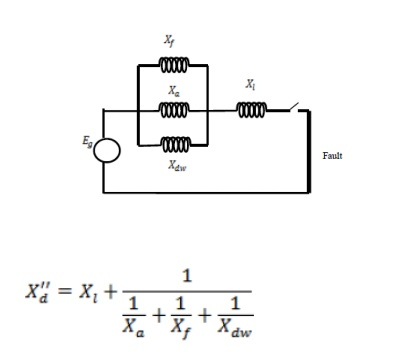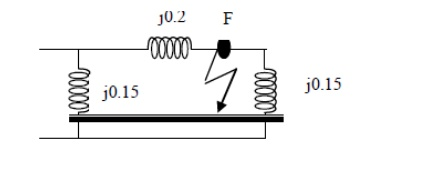Home | | Power System Analysis | Symmetrical Fault

# Symmetrical Fault

In symmetrical faults all the three phases are short circuited to each other and to earth also.

SYMMETRICAL FAULT

In symmetrical faults all the three phases are short circuited to each other and to earth also. Such faults are balanced and symmetrical in the sense that the voltage and current of the system remains balanced even after the fault and it is enough if we consider any one phase

## Short circuit capacity of power system or fault level.

Short circuit capacity (SCC) or Short circuit MVA or fault level at a bus is defined as the product of the magnitude of the pre fault bus voltage and the post fault current## Synchronous reactance or steady state condition reactance

The synchronous reactance is the ratio of induced emf and the steady state rms current. It is the sum of leakage reactance (Xl) and the armature reactance (Xa).## Sub transient reactance

The synchronous reactance is the ratio of induced emf on no load and the sub transient symmetrical rms current.## Transient reactance

The synchronous reactance is the ratio of induced emf on no load and the transient symmetrical rms current.Fault current in fig., if the Pre-fault voltage at the fault point is 0.97 p.u.## Thevenin’s theorem:

(i). Fault current = Eth / (Zth+Zf)

(ii). Determine current contributed by the two generators IG1 = If * (Z2/(Z1+Z2))

IG2 = If * (Z1 / (Z1+Z2))

(iii). Determine Post fault voltage Vif = Vi°+ΔV = V°+(-Zi2*IGi)

(iv). Determine post fault voltage line flows      Iij = (Vi –Vj) / Zij series

(v). Short circuit capacity If = │Eth2 / Xth

Study Material, Lecturing Notes, Assignment, Reference, Wiki description explanation, brief detail
Power System Analysis : Fault Analysis : Balanced Faults : Symmetrical Fault |